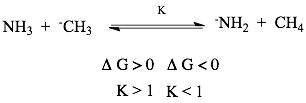# Problem: For the following reaction, indicate by circling the correct answers for  ΔG and K, whether this reaction lies to the right or left.

###### FREE Expert Solution
96% (353 ratings)
###### Problem Details

For the following reaction, indicate by circling the correct answers for  ΔG and K, whether this reaction lies to the right or left.Frequently Asked Questions

What scientific concept do you need to know in order to solve this problem?

Our tutors have indicated that to solve this problem you will need to apply the Chemical Equilibrium concept. If you need more Chemical Equilibrium practice, you can also practice Chemical Equilibrium practice problems.

What is the difficulty of this problem?

Our tutors rated the difficulty ofFor the following reaction, indicate by circling the correct...as high difficulty.

How long does this problem take to solve?

Our expert Organic tutor, Jonathan took 2 minutes and 43 seconds to solve this problem. You can follow their steps in the video explanation above.